cancel
Showing results for
Did you mean:Level 1
7 5 0 0
Message 1 of 6
710
Flag Post

Solved!

# adding units on a resultHP Recommended
hp prime

in the 1980s with the HP41 CV i habve been able to add units to the calc result like

speed=120 km/h

is there a way to do it the same way with the new PRIME calculator

thanks for any help

have a nice day

br

may be i have to point out, that i try to solve this problem in  the DEFINE mode

so, i define a fromula and i would like to add units to the result

sorry & thanks

Tags (1)
1 ACCEPTED SOLUTION

Accepted SolutionsLevel 5
115 114 26 45
Message 4 of 6
Flag PostHP Recommended

Hi Atschlwi,

Firstly, I need to know what your formula is and the units involved in the equation.

i.e 1MJ = 1000kJ = 1000kg*m^2/s^2(SI unit)=1MWatt.s(Watt-Seconds)=238.845896627kcal (Kilo Calories)

The equation
0.488*G^0.75*1_(MJ CE/kg) What is G? and MJ (Prime does not know that MJ is Megajoule)
so, it should be typed in as 1000000_J or 10^6_J.. CE(Conversion Energy?) does not stand for anything as Prime does not know What units (or SI units) the CE is comprised of.

Here are some of Energy units that Prime understands: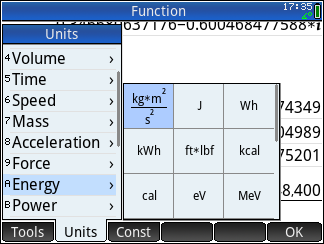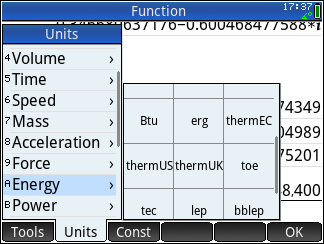Here are picture of some conversions Prime can do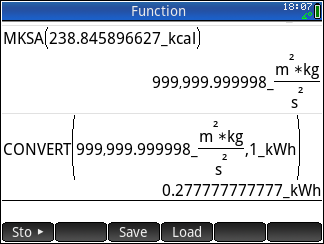If you can give me more information of the units that are involved in your equation e.g Acceleration =20_(m/s)/2_(s)=10_(m/s^2) .

Tags (1)
5 REPLIES 5Level 5
115 114 26 45
Message 2 of 6
Flag PostHP Recommended

Hi Atschlwi,

If you already have a formula defined as Function then you just need to modify the equation and add *1_(kph). i.e. Multiply the final result with 1 unit measure.

To modify the Defined function Select Shift toolbox(Mem) to get into Memory Manager -> Select User Variables then View Softkey -> then Scroll down until your Defined Function name appears -> Highlight your function then Select Edit Soft Key. At the end of your equation enter the following *1_(kph) the Ok to save the function then Esc to return to home mode.

My simple example here is Defined Function name: SPEED and Formula is A*B*1_(kph)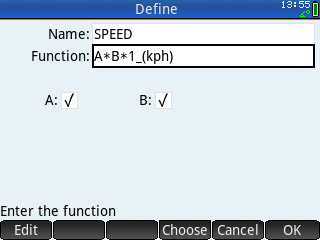To use my example function in Text mode or CAS mode is SPEED(67,23)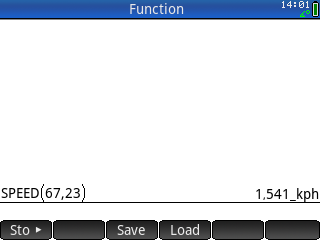Hope this helps.Level 1
7 5 0 0
Message 3 of 6
Flag PostHP Recommended

hi corruptissima

thank you very much for your hints

is it possible, that the calculater accepts only some how standard units.

will you pleasse figure it out for >>

mega joule convertible energy per kg weight

MJ CE / kg

0,488*G^0,75*1_(MJ CE/kg)

it doesn´t work

if i ad 1_(kph) >>> it works !!!!

thanxsLevel 5
115 114 26 45
Message 4 of 6
Flag PostHP Recommended

Hi Atschlwi,

Firstly, I need to know what your formula is and the units involved in the equation.

i.e 1MJ = 1000kJ = 1000kg*m^2/s^2(SI unit)=1MWatt.s(Watt-Seconds)=238.845896627kcal (Kilo Calories)

The equation
0.488*G^0.75*1_(MJ CE/kg) What is G? and MJ (Prime does not know that MJ is Megajoule)
so, it should be typed in as 1000000_J or 10^6_J.. CE(Conversion Energy?) does not stand for anything as Prime does not know What units (or SI units) the CE is comprised of.

Here are some of Energy units that Prime understands:Here are picture of some conversions Prime can doIf you can give me more information of the units that are involved in your equation e.g Acceleration =20_(m/s)/2_(s)=10_(m/s^2) .

Tags (1)Level 1
7 5 0 0
Message 5 of 6
Flag PostHP Recommended

hi there

the formula calulates

convertible energy in mega joule  per kg weight (G) of a cow

CE                                  MJ               /            kg

0,488 >> constant

CE =0,488*G^0,75*1_(MJ/kg)

so meanwhile i guess i could solve the issue

by simply removing then convertible energy CE out of the unit

because this should be clear some how anyways

again

thank you very much for your detailed explanation.

my be i´ll take a course on using the calculator one day

ha ha

have a nice one

BRLevel 5
115 114 26 45
Message 6 of 6
Flag PostHP Recommended

HI Atschlwi,

Maybe this is the what you're looking for.

Only if Constant has value .488/Kg^2 the G is mass of a Cow in kg.

Then the equation CE is 0.488_(kg^(−2))*500^0.75*1_(kg)*1000000_(J)

so the Defined function looks like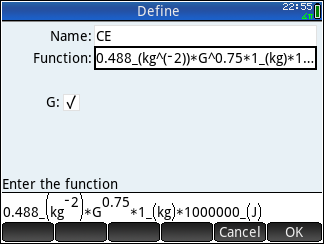And solution is shown in joule so you need to remove 1E6_(J) for megajoule answer.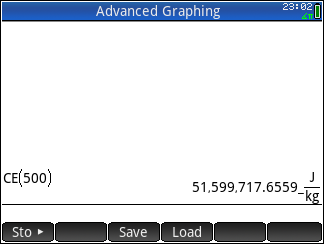As there is no way to specify the MJ in prime other than programing it you have to convert the answer divide by 1E6.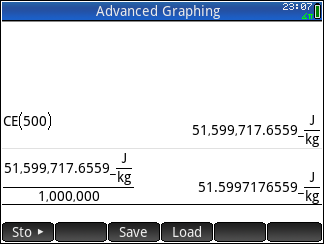So the answer is 51.59971756559 MJ/kg. Alternatively, You can remove 1E6 in this equation since you know that answer is in terms of Megajoule/Kilo i'e 0.488_(kg^(−2))*500^0.75*1_(kg)*1_(J), But, the catch is that if you use the program later with the last equation, you might not remember if the answer should be in megajoules.

Here is a short program of calculation of CE value in MJ/kg. How ever the answer is returned in String so you cannot use it for direct number calculation.Program listing, So you can copy into the virtual Prime to test:

// Calculate Coversion Energy
// of MJ/kg of Per kilo of cow
EXPORT CE(G) // Function name
BEGIN
LOCAL ce; // Declaration of local value
ce:=0.488*G^.75; // Formular